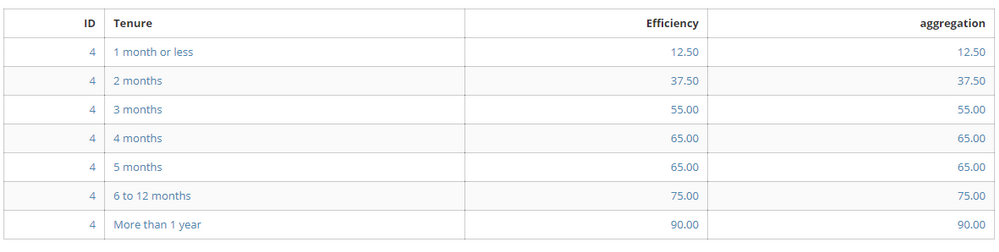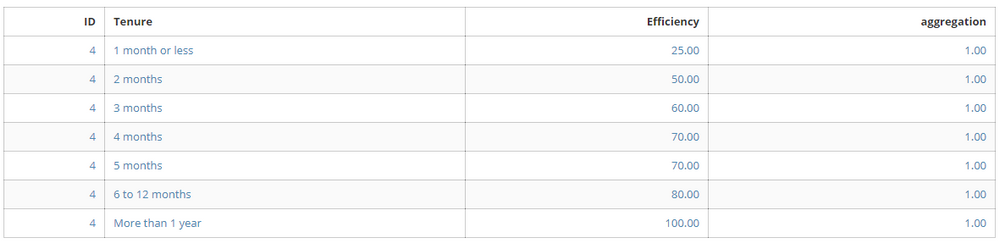# Aggregating a column in Beast Mode

Is it possible to aggregate an entire column in a beast mode calculation within analyzer? (I have found videos explaining this within a SQL ETL, but not as a calculated field in analyzer).

For example: In the table below I want to divide the value in the "efficiency" column by the averge of all values in the column. Right now, the "aggregation" column is a calculated field with the formula =AVG('Efficiency'). It returns the value in the "Efficiency" column, so if I were to divide, I would always get "1." But I want each efficiency value divided by 57.14 - which is the average of all efficiency values.Is this possible with a Beast mode calculation, or do I need to edit the ETL? If I do need to edit the ETL, the card using this aggregation is the third frame of a drill path. Will the path be affected?

Thanks for the help!

Tagged:

•CoachWithin beast mode this isn't possible because you can only do a single group level. Do display the single values and then get the sum of those values would require two separate grouping levels (individual values and entire dataset level). It's possible with a subquery in SQL but not possible in a beast mode.

**Did this solve your problem? Accept it as a solution!**

• Hi @rgbuckley,

The calculation below should do the trick:

'Efficiency' / AVG('Efficiency')

Let me know how that goes

Cheers,

Eric

• Hi Eric,

Thanks for reacing out. When I do the calculation you suggest, the calculated field always returns 1, since it is taking the efficiency value divivded by the average of that one efficiency value. I need to aggregate the column before dividing. Thoughts?•Coach